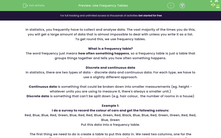# Use Frequency Tables

In this worksheet, students will practise using frequency tables to collect and sort dataKey stage:  KS 4

Year:  GCSE

GCSE Subjects:   Maths

GCSE Boards:   AQA, Eduqas, Pearson Edexcel, OCR,

Curriculum topic:   Statistics

Curriculum subtopic:   Statistics Interpreting and Representing Data

Difficulty level:#### Worksheet Overview

In statistics, you frequently have to collect and analyse data. The vast majority of the times you do this, you will get a large amount of data that is almost impossible to deal with unless you write it as a list.

To get round this, we use frequency tables.

What is a frequency table?

The word frequency just means how often something happens, so a frequency table is just a table that groups things together and tells you how often something happens.

Discrete and continuous data

In statistics, there are two types of data - discrete data and continuous data. For each type, we have to use a slightly different approach.

Continuous data is something that could be broken down into smaller measurements (eg. height - whatever units you are using to measure it, there's always a smaller unit.)

Discrete data is something that can't be split down (e.g. hair colour,  the number of rooms in a house)

Example 1:

I do a survey to record the colour of cars and get the following colours:

Red, Blue, Blue, Red, Green, Blue, Red, Red, Blue, Green, Red, Black, Blue, Blue, Red, Green, Green, Red, Red, Blue, Green

Put this data into a frequency table.

The first thing we need to do is create a table to put this data in. We need two columns, one for the category (in this case colour) and one for the frequency (how many times we get this colour).

 Colour Frequency Red Blue Green Black

We now need to count how many of each colour we have, and this will give us the following, complete frequency table:

 Colour Frequency Red 8 Blue 7 Green 5 Black 1

Example 2:

I measure the heights of some children and get the following results (all given in centimetres):

132, 145, 165, 133, 142, 147, 152, 161, 164, 131, 135, 137, 149, 148, 171, 140, 150

Put this data into a grouped frequency table.

This one is slightly different - we can't use exactly the same approach as before because all the numbers are different. This will usually happen if you are measuring something that can be measured on a scale, such as  heights or weights.

To deal with this, we use a grouped frequency table. The difference between this and a frequency table is that instead of having one thing for the category, we have a range.

 Height (cm) Frequency 130 < h ≤ 140 140 < h ≤ 150 150 < h ≤ 160 160 < h ≤ 170 170 < h ≤ 180

Let's take a couple as examples.

The first number is 132, which would clearly go into the 130 < h ≤ 140 box.

The last number is 150 -  you have to think about  this a bit more. 140 < h ≤ 150 means anything up to and including 150, whereas  150 < h ≤ 160 means anything above 150.

150 would go into the 140 < h ≤ 150 box.

 Height (cm) Frequency 130 < h ≤ 140 6 140 < h ≤ 150 6 150 < h ≤ 160 1 160 < h ≤ 170 3 170 < h ≤ 180 1

Time for some questions now.

### What is EdPlace?

We're your National Curriculum aligned online education content provider helping each child succeed in English, maths and science from year 1 to GCSE. With an EdPlace account you’ll be able to track and measure progress, helping each child achieve their best. We build confidence and attainment by personalising each child’s learning at a level that suits them.

Get started